# An Efficient Hybrid DC to DC Boost Converter with Bi-Polar Symmetrical Voltage Multiplier for Renewable Applications

London Journal of Engineering Research
Volume | Issue | Compilation
Authored by Srinivas Singirikonda , N.Neelima
Classification: NA
Keywords: DC-DC converter, renewable energy sources, voltage multiplier, interleaved structure.
Language: English

In this paper implementation, operation and design analysis of the hybrid boost DC-DC converter is described for renewable energy sources andlow voltage sources. Here the Combination of interleaved structure of inductor and voltage multiplier structure are implemented. Open loop control and closed loop fuzzy control of this converter is employed in the MATLAB SIMULINK and output voltage waveforms are shown.

# An Efficient Hybrid DC to DC Boost Converter with Bi-Polar Symmetrical Voltage Multiplier for Renewable Applications

## ____________________________________________

1. ### ABSTRACT

In this paper implementation, operation and design analysis of the hybrid boost DC-DC converter is described for renewable energy sources and low voltage sources. Here the Combination of interleaved structure of inductor and voltage multiplier structure are implemented. Open loop control and closed loop fuzzy control of this converter is employed in the MATLAB SIMULINK and output voltage waveforms are shown.

Keywords: DC-DC converter, Renewable energy sources, voltage multiplier, interleaved structure.

Author α: IEEE Member, Assistant Professor, TKRCET, Hyderabad, Telangana, India.

σ : IEEE Student Member, M.Tech (power electronics) scholar TKRCET, Hyderabad, Telangana, India.

1. ### INTRODUCTION

Nowadays the renewable energy systems are expecting the generation of high voltage gain DC-DC converters with high efficiency and low cost, so DC-DC converter is good for economical point of view and reliable to loads. The front end PV system generally requires and wants the step-up (boost) converter which has the characteristics of boosting the voltage with good regulation capability. PV system and renewable energy sources have low and variable terminal voltages, So DC-DC converter is placed between PV systems or renewable energy sources with HVDC (high voltage DC) applications . Fuel cell energy system also requires high gain DC-DC converter because it has low voltage at output side (storage side). Actually, conventional DC converters can offer limited gain and their efficiency reduces as the gain requirement increases. Switched capacitor structure, tapped or coupled inductor based technique, transformer based techniques, voltage multiplier structures are implemented in recent years. So each method has its own advantages and limitations. Switched capacitor DC-DC power converters are used a network of switches and capacitors. This switched capacitor converters are ideal for power electronic applications . It enables high efficiency and it can be used in number of applications in the power conversion space. But it has poor regulation capability and high pulsating current. The DC-DC boost converter is used to convert the unregulated DC input to a controlled DC output at a desired voltage level. They generally perform by applying a DC voltage across an inductor or transformer a period of time usually in the range of 20KHZ to 5MHZ . It can store the energy in magnetic form and transferred across the output side in a controlled manner. So, it can be used in vehicles, solar powered uninterrupted power supplies and significant use in renewable energy sources. But it gives more circuit complexity and requires snubber circuit . So high boost DC-DC converter with hybrid transformer method is presented to achieve high output voltage with high efficiency. The boost DC-DC converters have been proposed to obtain high voltage without extreme high duty cycle by using isolated transformer or coupled inductor which are discussed previously. The design of the high efficiency transformers, coupled inductors or resonant components for these converters are relatively complex, compared with the proposed boost DC-DC converter . By cascading diode-capacitor or diode-inductor modules, these kinds of DC-DC converters provide not only high voltage gain but also simple and robust structure. The proposed Cockcroft Walton voltage multiplier is very popular among high voltage than that of the conventional Cockcroft Walton voltage multiplier . The problems in previous methods are poor regulation capability, switching losses, less voltage gain, poor efficiency, more voltage ripple, less voltage gain, more component stress and circuit complexity . So, the design of boost DC-DC converter will make according to their application. In this paper, the new hybrid boost converter with single switch and single inductor is proposed by employing the bipolar voltage multiplier with interleaved structure to get the high voltage gain and efficiency.

1. ### IMPLEMENTATION OF PROPOSED METHOD

In this chapter implementation of proposed method is described as follows in below fig: 1.The input of solar charge controller or battery is given by photo voltaic cells or renewable energy sources. Charge is controlled at this stage and it is stored in the battery and it is given to the DC-DC boost converter as voltage source. DC-DC boost converters are developed to get the high voltage and efficiency . Different type of DC-DC boost converter methods are achieved and implemented to get great efficiency. The output of this converter is given to various applications like DC loads and low voltage sources.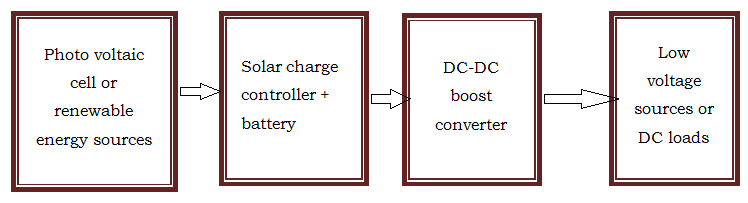Fig. 1: Block diagram of proposed method.

In further chapter, design and explanation of DC-DC boost converters are explained.

1. ### DESIGN AND OPERATION

Design and operation of hybrid boost converter explained by inductive core switching interlaced structure and bipolar voltage multiplier.

a) Inductive core switching:  The combination of input source voltage, inductor and MOSFET switch perform as a main role. The inductive core switching is shown in fig: 2. It can give two opposite or relative PWM voltages at port AO and port OB. Although the two voltage waveforms have their individual high voltage level and low voltage level then the gap between two levels is similar to each other . The purpose of MOSFET switch is used in this converter to maintain less switching losses. This Switch is changing the duty ratio according to Ton, T off.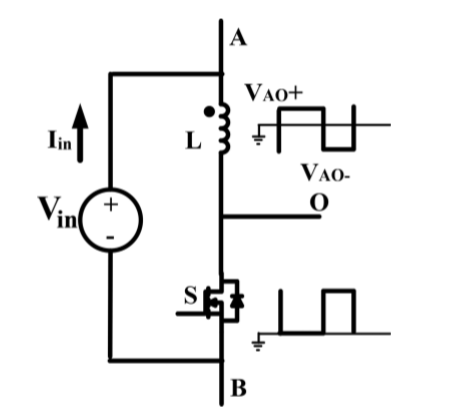Fig. 2: Design of Inductive core switch.

b) Interleaved structure: Nowadays the interlaced high boost DC-DC converter has been widely used in photo voltaic generators, electric vehicles and power factor correction due to its high power density . Interlaced connection is a method in which the additional circuit is added in between the input and output of the main circuit in order to achieve high efficiency and output voltage of the converter . Interlaced parallel structure has been applied in many high power density applications, in order to minimize the input current ripple, reduce the circuit size, and improve the power level. This interleaved structure of hybrid boost converter consists of two boosting sections to achieve high gain without extreme duty ratio .

c) Bipolar voltage multiplier: It is the main structure of the hybrid boost converter with single switch. Hence the operation of circuit is depending upon the bipolar voltage multiplier. It means that the positive and negative multipliers are to be implemented to get the required output voltage and high gain with high efficiency . Bipolar voltage multiplier is composed of two branches, positive multiplier and Negative multiplier.Fig. 3: The design of  proposed hybrid boost converter.

Here positive multiplier is same as that of the traditional voltage multiplier while negative multiplier has the input at the cathode terminal of cascaded diodes, which can generate negative voltage at anode terminal . By defining the high voltage level at AO as VOA+, the low voltage level as VOA- and the duty cycle of high voltage level is D, and low-level voltage is 1-D.

d) Ratings of hybrid boost converter: The below table is given the ratings of the hybrid boost converter.

Table 1: Ratings of proposed hybrid boost converter.

 S.no Parameter Value 1. Switching frequency, input voltage 40KHZ, 50V 2. Duty ratio 0.5 3. Time period 25μs 4. Resistor 722Ω 5. Inductor 100μF 6. Capacitor 500Μh 7. Diode 200V/20A, VF=0.78V (STH2002C)

e) Circuit operation: The capacitors are charging in parallel and discharging in series to get the output voltage greater than input voltage. So, during the operating mode the two capacitors are charged in parallel with other two capacitors either even or odd will be discharged in series . If the diode is conducting due to the input voltage, then the capacitor connected in parallel is charging and other capacitors are in discharging mode. The capacitor fully charged in parallel during switch on position of MOSFET then after diode is in off position automatically and parallel capacitor is in series with the series charging capacitor then voltages are to be added. So, this is the main process which is discussed in below.

Explanation of MOSFET switch is in on position:

In this mode, the total input voltage applied to the inductor terminal A and O nodes. Then this voltage appears across the output terminal including charging and discharging operation of capacitors and conducting of diodes takes place. So voltage across O and B points are to be zero and resistance of MOSFET is considered as negligible. At this mode, capacitor C2a is discharged.

Explanation of MOSFET Switch is in off position:

In this mode, the switch is connected to negative voltage across O and B, so that the voltage across A is to be zero. At this switching mode C2a will be charged.

Continuous and discontinuous modes: According to previous mode C2a will be charging from inductor L in reverse direction, C2b will be discharging through L. When the inductive current is discharging as the switch is in turned off position, then the current doesn’t touch to zero then it is called as continuous conduction mode.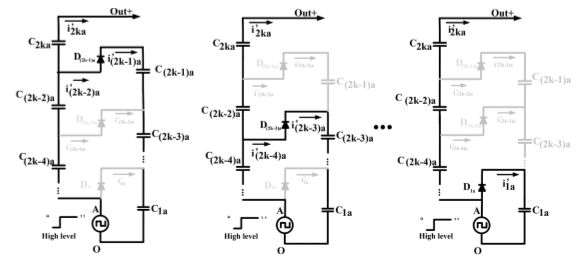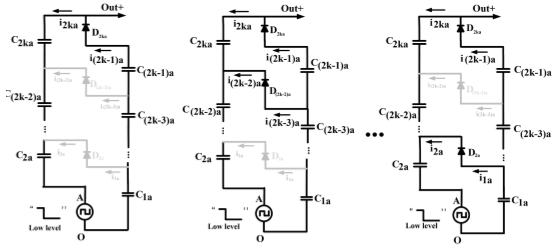Fig. 4: Analysis of Switch on position of MOSFET.

Fig. 5: Analysis of Switch off position of MOSFET.

In the discontinuous conduction mode, the current of inductor touches to zero, basically it is taken as a discrete system.

Calculation of capacitors involved in this circuit(1)((2)

K=6 for even, K=5 for odd series in this project.

D is considered as duty ratio. D=0.5.

C=3600UF as per calculation for even numbered capacitor, C=2100UF is for odd numbered capacitor.

For K=6, ThenC2a (equivalent) = C2b (equivalent) = 144UF.

C1b (equivalent) = C1a (equivalent) = 240UF.

In next section closed loop fuzzy logic control of hybrid boost converter circuit is implemented in MATLAB simulation to control the output voltage.

### V.    CLOSED LOOP FUZZY CONTROLLER TO HYBRID BOOST CONVERTER

Fuzzy logic control is automatic control technique it is closed loop control of any system. This is dealing with analog inputs like ‘0’ and ‘1’ and explains the rules, instructions by taking if and then sentences. In an electrical system, so many machines are controlled by this closed loop technique, because it gives controlled output and reliability of the machine. Rules are implemented to perform the operation and gives controlled output signal. Each variable consists of 7 membership functions along with OR, AND operations. NB=Negative B, NM=Negative medium, NS=Negative small, ZE=Zero, PS=Positive small, PM=Positive medium, PB=Positive B are compared according to 49 rule based fuzzy logic controller .

Table 2: Rules to fuzzy logic controller.

 Error /Change in Error NB NM NS EZ PS PM PB PB Z PS PM PB PB PB PB PM NS Z PS PM PB PB PB PS NM NS Z PS PM PB PB EZ NB NM NS Z PS PM PB NS NB NB NM NS Z PS PM NM NB NB NB NM NS Z PS NB NB NB NB NB NM NS Z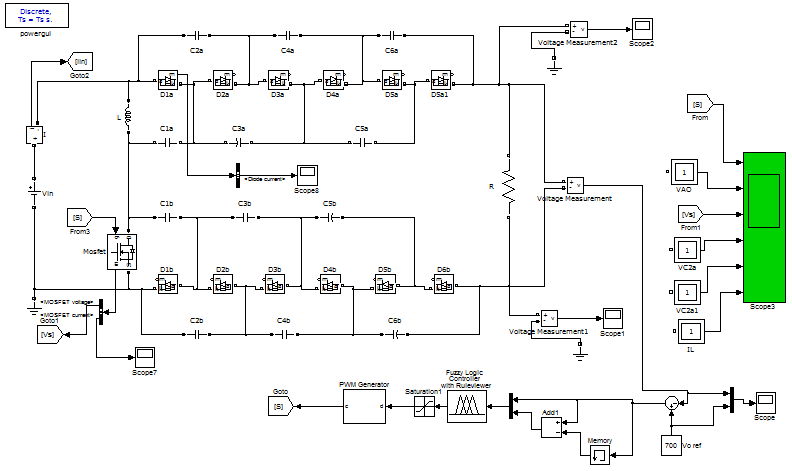Fig. 6: Fuzzy logic control circuits of hybrid boost converter circuit.

This control technique is connected to the output side with closed loop control parameters. Those are to be constructed by MATLAB SIMULINK. This is dealing with analog inputs like ‘0’ and ‘1’ and explains the rules, instructions by taking if and then sentences. In an electrical system, so many machines are controlled by this closed loop technique, because it gives controlled output and gives reliability of the machine. In next section, switching losses and efficiency and simulation results are shown.

1. ### SWITCHING LOSSES AND EFFICIENCY AND SIMULATION RESULTS

6.1  Switching losses

Switching losses are considered according to MOSFET in the main circuit. MOSFET has negligible resistance then on state losses are considered with current through MOSFET.

Here I = 31A is taken from output waveforms, and R = 0.01Ω.

Power loss of MOSFET = I2R = 9.61W.

Power loss of diode = I2R = 0.588 W.

Here I = 7A is taken from output waveform.

Total switching losses = Power loss of MOSFET + Power loss of diode.

Total switching losses = 9.61+0.588=10.198W.

By observing above losses, the proposed method is advantage for further application. And remaining all components have passive nature so no losses are represented. So, diode and MOSFET losses are taken as consideration.

6.2  Efficiency

From output voltage waveforms V o = 800v. So,=92.7%

So according to this, high efficiency is obtained. Simulation waveforms of hybrid boost converter with and without fuzzy logic controller is described as follows.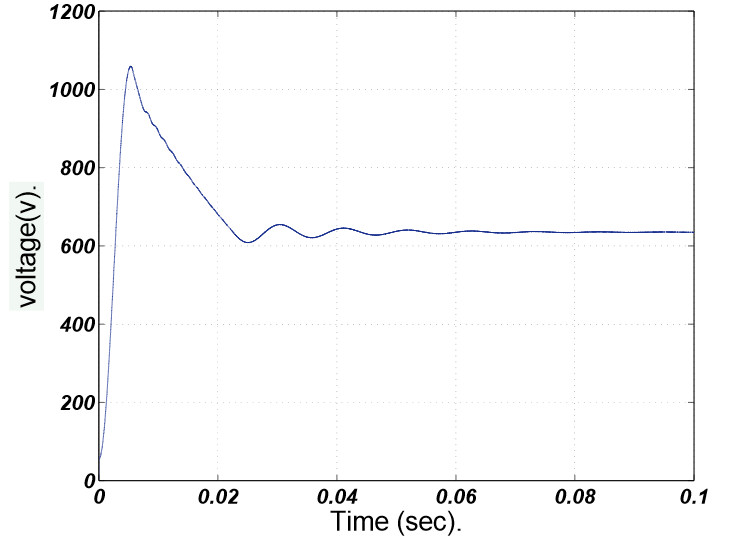Fig. 7: Simulation waveforms of converter without fuzzy logic controller.

The above result is plotted between output voltage and time. The settling time is 0.02sec to reach steady state value. The maximum peak overshoot value of this plot is 1090V before reached steady state.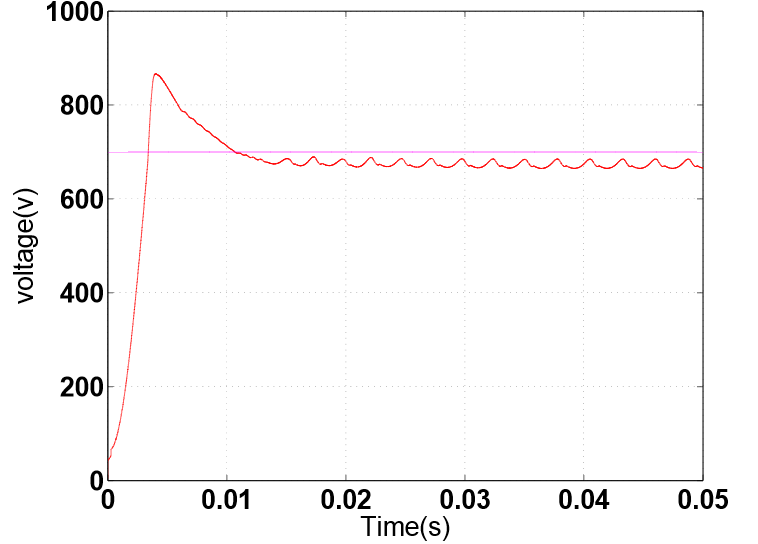Fig. 8: Simulation waveforms of converter with fuzzy logic controller.

The above result is plotted between output voltage and time. The settling time is 0.01sec to reach steady state value. The maximum peak overshoot value of this plot is 890V before reached steady state.

Table 3: Results comparison of hybrid boost converter according without and with fuzzy logic control.

 S.no Parameter Hybrid boost converter without fuzzy logic controller Hybrid boost converter with fuzzy logic controller 1. Peak overshoot(V) 1090 890 2. Output voltage(V) 620 690 3. Settling time(Sec) 0.02 0.01

Table 4: Comparison of duty ratios, output power and efficiency without, with controller fuzzy logic controller.

 S. No Duty ratio Power without fuzzy logic controller (w) Power with fuzzy logic controller (w) Efficiency without fuzzy logic controller (%) Efficiency with fuzzy logic controller (%) 1 0.5 532.40 659.41 91.8 92.7 2 0.6 864.40 931.3 93.6 93.9 3 0.7 1440.99 1895.9 95 95.7 4 0.8 3116.34 3634.9 96.6 96.9 5 0.9 5540.16 11648.19 97.5 98.2

By observing above results, hybrid boost converter with fuzzy logic controller attained the high steady state voltage than the without controller with in less time of 0.01sec, and peak overshoot decreased in hybrid boost controller with fuzzy logic controller as shown in table:3. And duty ratios, output power and efficiency are compared in table: 4.

Power and efficiency values are compared in below fig: 9, fig: 10 with and without controller.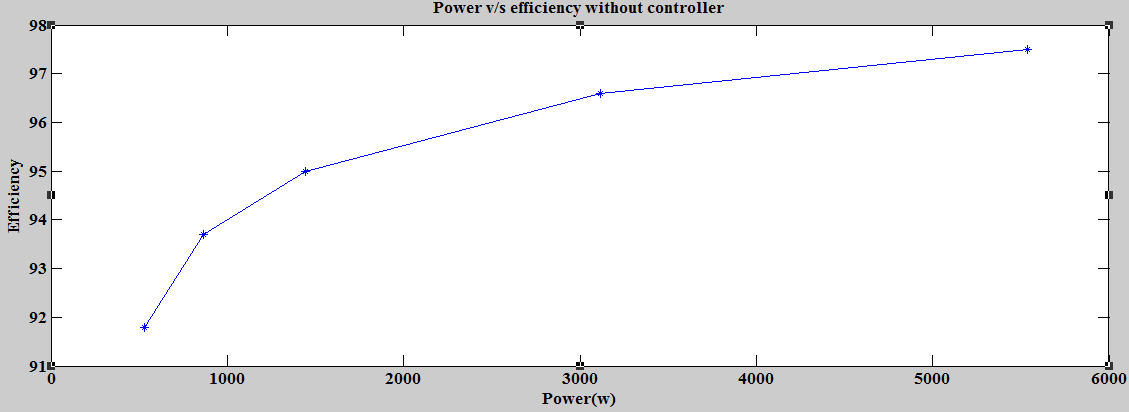Fig. 9: Power v/s efficiency without controller.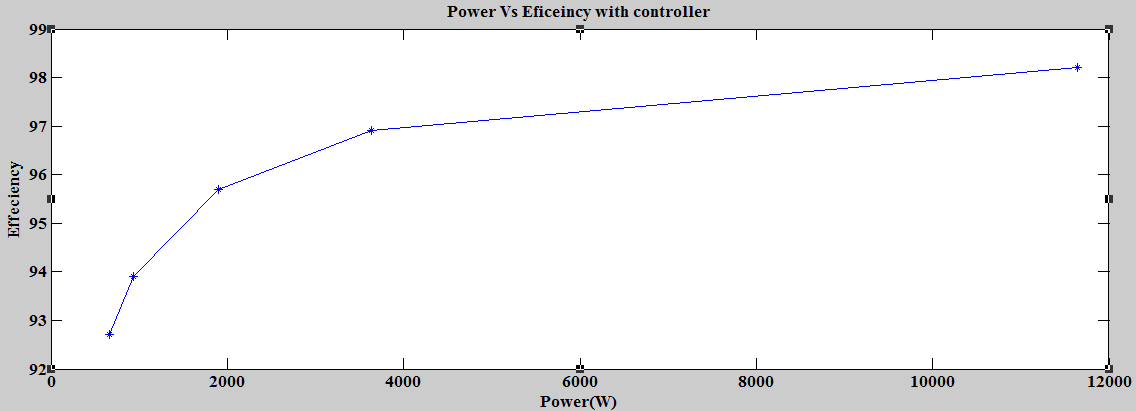Fig. 10: Power v/s efficiency with controller.

1. ### CONCLUSION

In this paper, a new hybrid boost converter is proposed with the inductive core switching structure, and combination of bipolar voltage multiplier structure and interleaved structure of hybrid boost converter is implemented. The proposed converter achieved efficiency of 92.7% and got voltage of 690V. The closed loop fuzzy logic control technique is implemented for hybrid boost converter to control the output voltage. Output waveforms of hybrid boost converter without and with fuzzy logic controller are compared and duty ratios, output power and efficiency are compared. The proposed hybrid boost converter is suitable for renewable energy application with above efficiency and voltage, such as the front end of PV system.

### REFERENCES

1. P. W. LEE, X. C. LIU, “Steady-state analysis of an interleaved boost converter with coupled inductors,” IEEE Trans. Ind. Electron., vol. 47, no. 4, pp. 787–795, Aug. 2000.
2. J. S. Brugler, “Theoretical performance of voltage multiplier circuits,” IEEE Journal of Solid-State Circuits, vol. 6, no. 3, pp. 132–135, June 1971.
3. G. ZHU and A. Ioinovici, “Switched- capacitor power supplies: dc voltage ratio, eﬃciency, ripple, regulation,” IEEE International Symposium on Circuits and Systems, pp. 553–556, May 1996.
4. LUNG-SHENG Yang and TSORNG-JUU Liang, “Novel high step up dc to dc converter with coupled inductor and switched capacitor for a sustainable,” IEEE Transactions on Industrial Electronics, Vol. 59, No. 1, January 2012.
5. R.J. WAI, R.Y. DUAN, “High step-up converter with coupled-inductor”, IEEE Transactions on Power Electronics, Vol. 20, No 5, September 2005. pp 1025-1035.
6. Yuan Mao Ye ;  K. W. E. Cheng  Sizhe Chen, “A High Step-up PWM DC-DC Converter With Coupled-Inductor and Resonant Switched- Capacitor”,  IEEE Transactions on Power Electronics (Volume: 32, Issue: 10, Oct. 2014) Page(s): 7739 – 7749, 29 November 2016.
7. Shenkman A. (2008) “A novel diode- capacitor voltage multiplier for increasing the voltage of photovoltaic cells,” in Proc. IEEE Compel, Zurich.
8. J. M. TOMBS, “An analog fuzzy logic controller for power converters”, THROUGH IEEE International Conference on Industrial Electronics, Control, Instrumentation (IECON 95), 1995, vol. 2, pp. 1490-1495.
9. H. X. LI and H. B. Gatland, “A New Methodology for Designing a Fuzzy Logic Controller”, IEEE Trans. On System, and Cybernetics, Vol. 25, No. 3, March 1995, pp. 505-512.
10. J.D. Lewis, H. R. Bolton, and N. W. Phillips, “Performance enhancement of single and two-phase SR drives using a capacitor boost circuit,” in European Power Electronics and Applications”, Conf. Rec., Vol. 3, pp. 229-232, 1995.
11. Pit-Leong Wong, Q. Wu, Peng XU, P. Yang, F. C. Lee, “Performance Improvements of Interleaving VRMs with coupling inductors”, IEEE Transactions on Power Electronics, 2001, pp. 499-507.
12. T. A. Meynard, H. Foch, P. Thomas, J. Courault, R. JAKOB, M. Nahrstaedt, “Multi cell Converters: Basic Concepts and Industry Applications”, IEEE Transactions on Industrial Electronics 2002, vol.49, no.5, pp. 955-964.
13. M. Prudente, L. L. Pfitscher, G. Emmendoerfer, E. F. Romaneli, and R. Gules, “Voltage multiplier cells applied to converters, non-isolated DC–DC,” IEEE Trans. Power Electron., vol. 23, no. 2, pp. 871–887, Mar. 2008.
14. J. Wang and P. Luerkens, “Complete model of parasitic capacitances in a cascade voltage multiplier in the high voltage generator,” in Proc. IEEE ECCE Asia Down under, Delft, 2013, pp. 18-24.
15. S. IQBAL, “A hybrid symmetrical voltage multiplier,” IEEE Trans. Power Electronics, vol. 29, no.1, pp. 18-24, Jan. 2013.
16. Srinivas Singirikonda, "Transient Stability of A.C Generator Controlled by Using Fuzzy Logic Controller" Int. Journal of Engineering Research and Applications ISSN:  2248-9622, Vol. 4, Issue 3 (Version 1), March 2014, pp. 389-395.

### Latest fromSrinivas Singirikonda#### For Authors

/symposiums/webinars, networking opportunities, and privileged benefits.
Authors may submit research manuscript or paper without being an existing member of LJP. Once a non-member author submits a research paper he/she becomes a part of "Provisional Author Membership".

Know more#### For Institutions

Society flourish when two institutions come together." Organizations, research institutes, and universities can join LJP Subscription membership or privileged "Fellow Membership" membership facilitating researchers to publish their work with us, become peer reviewers and join us on Advisory Board.

Know more#### For Subscribers

Subscribe to distinguished STM (scientific, technical, and medical) publisher. Subscription membership is available for individuals universities and institutions (print & online). Subscribers can access journals from our libraries, published in different formats like Printed Hardcopy, Interactive PDFs, EPUBs, eBooks, indexable documents and the author managed dynamic live web page articles, LaTeX, PDFs etc.

Know more